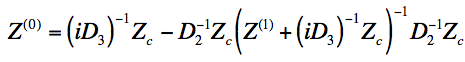Back to Kemp Acoustics HomeNext: Method for calculation of Up: Method for calculation of Previous: Projection across a discontinuity   Contents

## Projection along a cylinder

Substituting (2.39) and (2.43) into (2.95) gives an equation for the impedance at the left hand side of a uniform section of duct in terms of the impedance at the right hand side, being the multimodal version of equation (2.13):(2.99)

Now if we simplify by multiplying top and bottom by, (N.B. This is a correction to the original version of my thesis)(2.100)

Here(2.101)

The input impedance matrix may then be calculated from the output end impedance matrix by projecting alternately along a cylinder using equation (2.100) and across a discontinuity using equation (2.97) until the input end is reached.

Typically the acoustic horns that we will consider are cylindrical at the input end and only flare out towards the open end. The radiation impedance matrix at an open end is the subject of the next chapter. A waveguide terminated in an infinite cylindrical pipe is also a useful subject of theoretical study. In such an infinite terminating pipe all waves are forward going and the impedance is simply given by the characteristic impedance matrix of equation (2.42).

Back to Kemp Acoustics HomeNext: Method for calculation of Up: Method for calculation of Previous: Projection across a discontinuity   Contents
Jonathan Kemp 2003-03-24